# Create a Pipeline in Pandas

• Last Updated : 27 Oct, 2021

Pipelines play a useful role in transforming and manipulating tons of data. Pipeline are a sequence of data processing mechanisms. Pandas pipeline feature allows us to string together various user-defined Python functions in order to build a pipeline of data processing. There are two ways to create a Pipeline in pandas. By calling .pipe() function and by importing pdpipe package.

Through pandas pipeline function i.e. pipe() function we can call more than one function at a time and in a single line for data processing. Let’s understand and create a pipeline by using the pipe() function.

Attention geek! Strengthen your foundations with the Python Programming Foundation Course and learn the basics.

To begin with, your interview preparations Enhance your Data Structures concepts with the Python DS Course. And to begin with your Machine Learning Journey, join the Machine Learning - Basic Level Course

Below are various examples that depict how to create a pipeline using pandas.

Example 1:

## Python3

 `# importing pandas library``import` `pandas as pd` `# Create empty dataframe``df ``=` `pd.DataFrame()` `# Creating a simple dataframe``df[``'name'``] ``=` `[``'Reema'``, ``'Shyam'``, ``'Jai'``,``              ``'Nimisha'``, ``'Rohit'``, ``'Riya'``]``df[``'gender'``] ``=` `[``'Female'``, ``'Male'``, ``'Male'``,``                ``'Female'``, ``'Male'``, ``'Female'``]``df[``'age'``] ``=` `[``31``, ``32``, ``19``, ``23``, ``28``, ``33``]` `# View dataframe``df`

Output: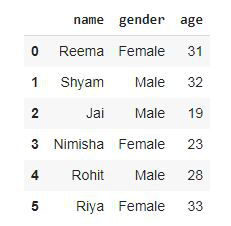Now, creating functions for data processing.

## Python3

 `# function to find maen``def` `mean_age_by_group(dataframe, col):``  ` `    ``# groups the data by a column and``    ``# returns the mean age per group``    ``return` `dataframe.groupby(col).mean()``  ` `# function to convert to uppercase``def` `uppercase_column_name(dataframe):``  ` `    ``# Converts all the column names into uppercase``    ``dataframe.columns ``=` `dataframe.columns.``str``.upper()``    ` `    ``# And returns them``    ``return` `dataframe `

Now, creating a pipeline using .pipe() function.

## Python3

 `# Create a pipeline that applies both the functions created above``pipeline ``=` `df.pipe(mean_age_by_group, col``=``'gender'``).pipe(uppercase_column_name)` `# calling pipeline``pipeline`

Output: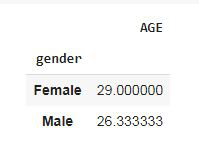Now, let’s understand and create a pipeline by importing pdpipe package.

The pdpipe Python package provides a concise interface for building pandas pipelines that have pre-conditions. The pdpipe is a pre-processing pipeline package for Python’s panda data frame. The pdpipe API helps to easily break down or compose complex-ed panda processing pipelines with few lines of codes.

We can install this package by simply writing:

`pip install pdpipe`

Example 2:

## Python3

 `# importing the package``import` `pdpipe as pdp``import` `pandas as pd` `# creating a emplty dataframe named dataset``dataset ``=` `pd.DataFrame()` `# Creating a simple dataframe``dataset[``'name'``] ``=` `[``'Reema'``, ``'Shyam'``, ``'Jai'``,``                   ``'Nimisha'``, ``'Rohit'``, ``'Riya'``]` `dataset[``'gender'``] ``=` `[``'Female'``, ``'Male'``, ``'Male'``,``                     ``'Female'``, ``'Male'``, ``'Female'``]` `dataset[``'age'``] ``=` `[``31``, ``32``, ``19``, ``23``, ``28``, ``33``]` `dataset[``'department'``] ``=` `[``'Accounts'``, ``'Management'``,``                         ``'IT'``, ``'IT'``, ``'Management'``,``                         ``'Advertising'``]` `dataset[``'index'``] ``=` `[``1``, ``2``, ``3``, ``4``, ``5``, ``6``]` `# View dataframe``dataset`

Output: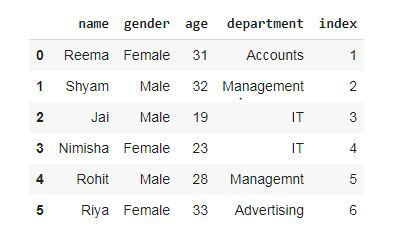Removing a column from dataframe using pdpipe.

## Python3

 `# creating a pipeline and``# droping the unwanted column``dropCol ``=` `pdp.ColDrop(``"index"``).``apply``(dataset)` `# display the new dataframe``# after column drop``dropCol`

Output: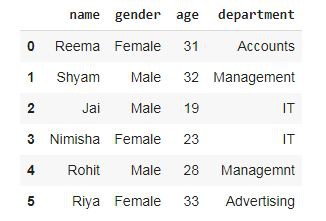There is another way to drop columns through pdpipe.

## Python3

 `# creating a pipeline and``# droping the unwanted column``dropCol2 ``=` `pdp.ColDrop(``"index"``)` `# applying the ColDrop to dataframe``df2 ``=` `dropCol2(dataset)` `# display dataframe``df2`

Output:Here, the column is dropped in two steps. In the first step, we created a pipeline and in the second step, we applied it to the dataframe.

#### Example 3:

Now we are adding one column to dataframe using pdpipe.

## Python3

 `# importing the package``import` `pdpipe as pdp``import` `pandas as pd` `# function to assign``# senior and junior in post``def` `fun(x):``    ``if` `x > ``30``:``        ``return` `"Senior"``    ``else``:``        ``return` `"Junior"`  `# creating a emplty dataframe named dataset``dataset ``=` `pd.DataFrame()` `# Creating a simple dataframe``dataset[``'name'``] ``=` `[``'Reema'``, ``'Shyam'``, ``'Jai'``,``                   ``'Nimisha'``, ``'Rohit'``, ``'Riya'``]` `dataset[``'gender'``] ``=` `[``'Female'``, ``'Male'``, ``'Male'``,``                     ``'Female'``, ``'Male'``, ``'Female'``]` `dataset[``'age'``] ``=` `[``31``, ``32``, ``19``, ``23``, ``28``, ``33``]` `dataset[``'department'``] ``=` `[``'Accounts'``, ``'Management'``,``                         ``'IT'``, ``'IT'``, ``'Management'``,``                         ``'Advertising'``]` `dataset[``'index'``] ``=` `[``1``, ``2``, ``3``, ``4``, ``5``, ``6``]` `# creating new column``# comparing with another column``# and applying the function``dataset[``'post'``] ``=` `dataset[``'age'``].``apply``(fun)` `# display dataframe``dataset`

Output: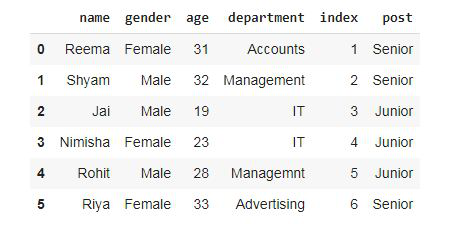Now, dropping the values from dataframe.

## Python3

 `#droping the values using ValDrop``df3 ``=` `pdp.ValDrop([``'IT'``],``'department'``).``apply``(dataset)` `#display dataframe``df3`

Output: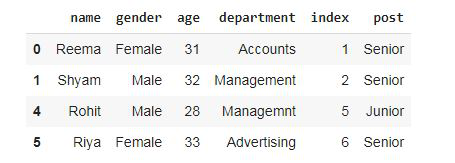The row containing ‘ IT ‘ value is dropped.

My Personal Notes arrow_drop_up